Function Repository Resource:

# PolyhedronFaceReflect

Reflect a polyhedron over a given face

Contributed by: Ed Pegg Jr
 ResourceFunction["PolyhedronFaceReflect"][polyhedron,k] reflects polyhedron over its kth face.

## Details

The polyhedron can be given as a Polyhedron object containing a list of vertices and a list of vertex indices.

## Examples

### Basic Examples (3)

In the octahedron given below, a list of vertices is followed by a list of faces (vertex indices):

 In:=Reflect the octahedron over its first face:

 In:=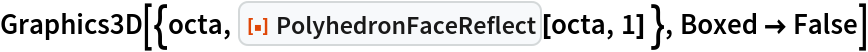Out=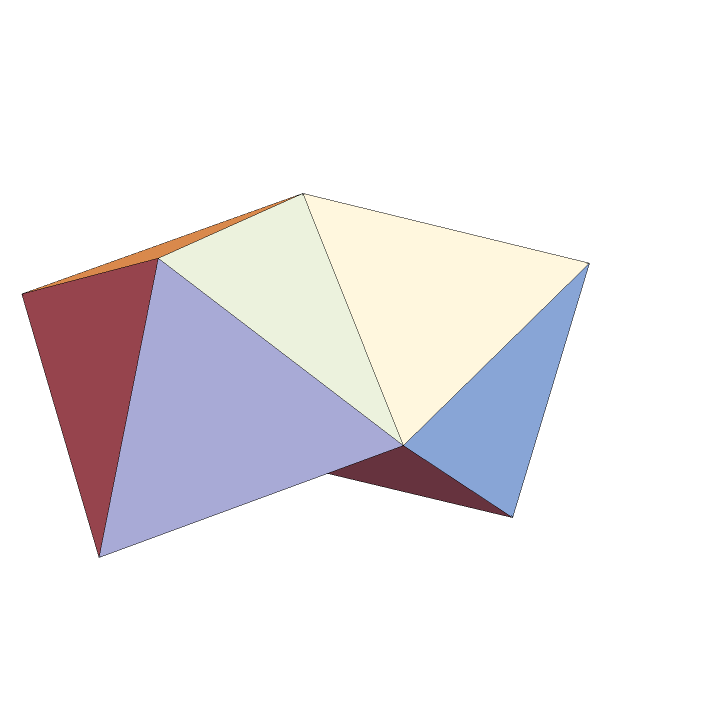Make a ring of eight octahedra:

 In:=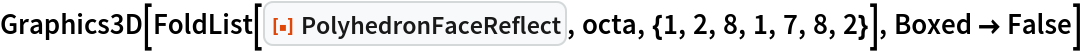Out=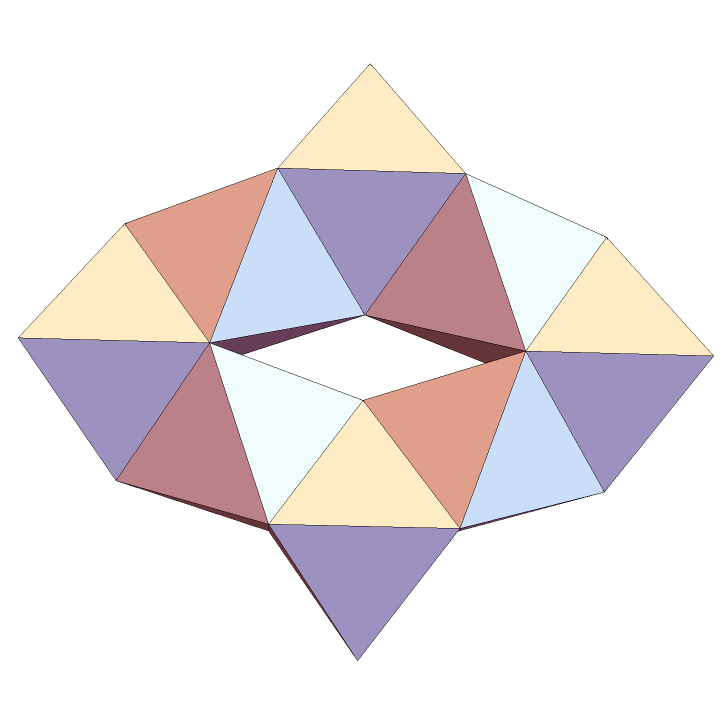### Scope (2)

Reflect a dodecahedron over its first face:

 In:=Out=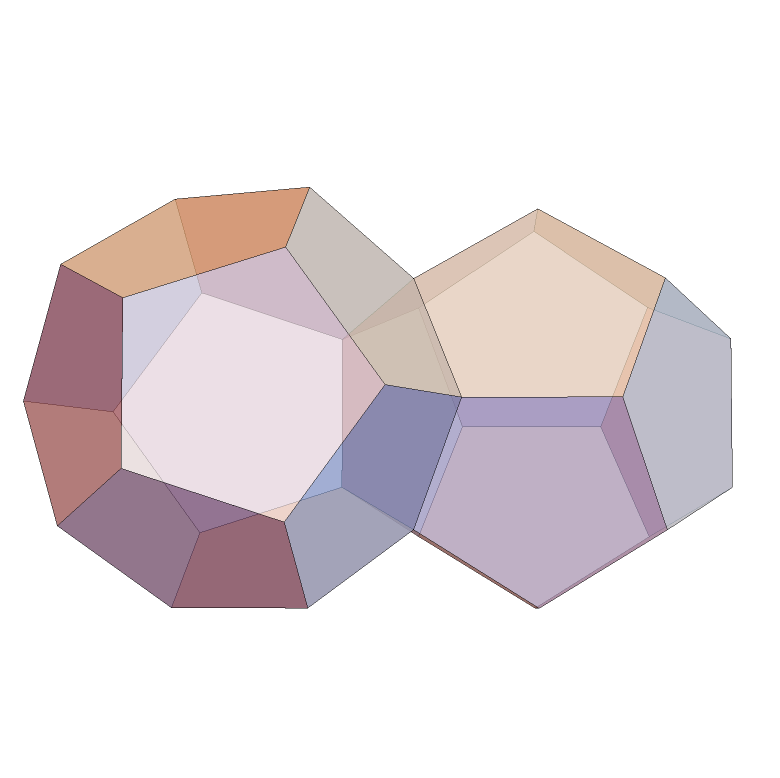Reflect the snub disphenoid over its fifth face:

 In:=Out=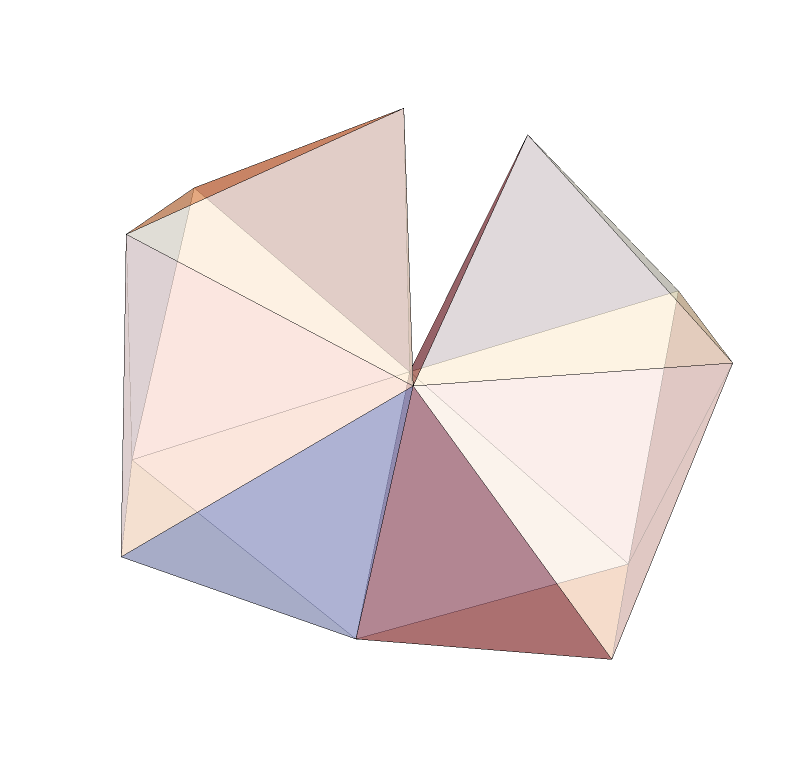### Neat Examples (3)

Make a perfect ring of twelve 4-antiprisms:

 In:=Out=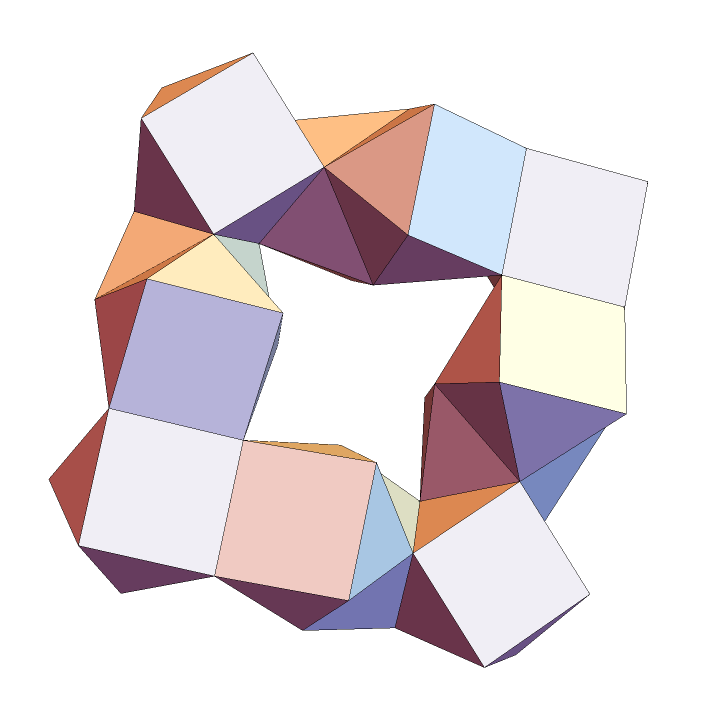Make a spiral of tetrahedra (the Boerdijk–Coxeter helix):

 In:=Out=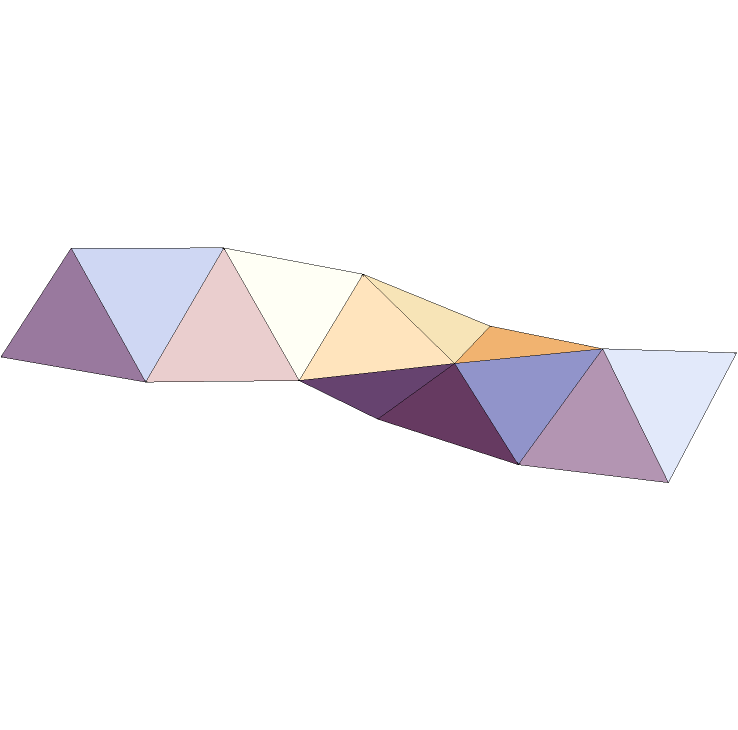Compare with the result of TetrahelixMesh:

 In:=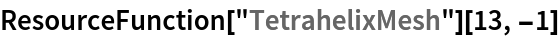Out=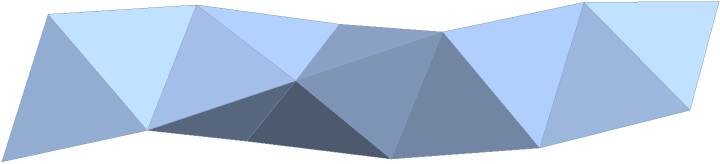Make a chain of icosahedra:

 In:=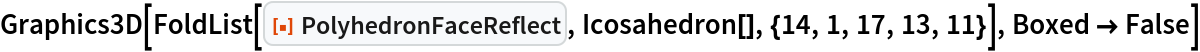Out=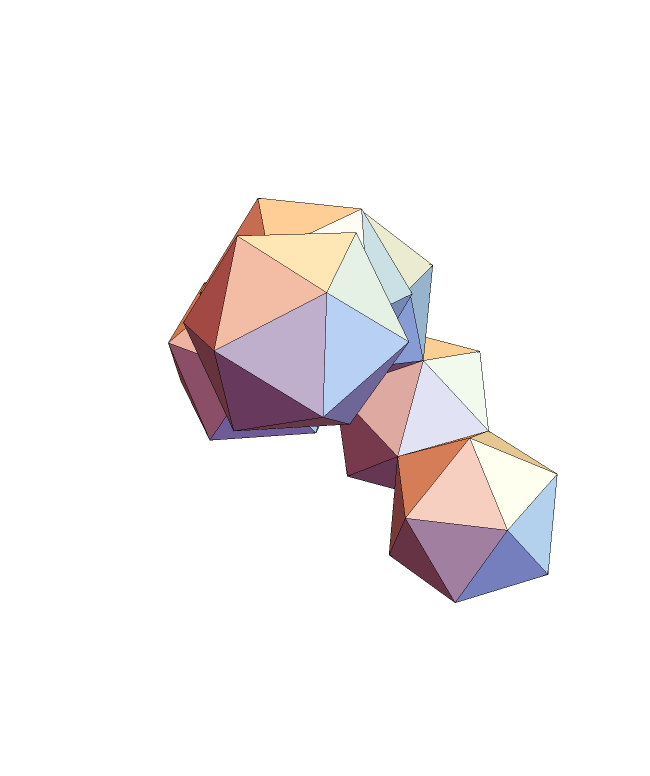## Version History

• 1.0.0 – 31 January 2022